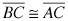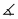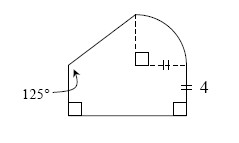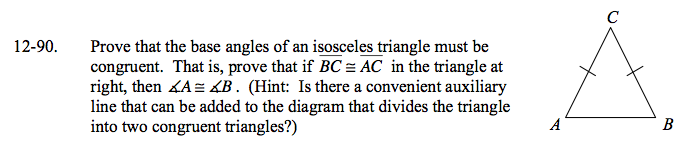### Home > GC > Chapter 12 > Lesson 12.2.4 > Problem12-90

12-90.

Prove that the base angles of an isosceles triangle must be congruent. That is, prove that ifin the triangle at right, thenA ≅B. (Hint: Is there a convenient auxiliary line that can be added to the diagram that divides the triangle into two congruent triangles?) Homework Help ✎